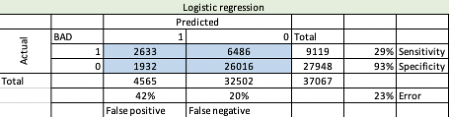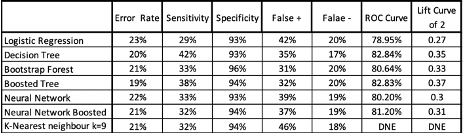top of page
Search

# Logistic Regression, Decision Tree, Bootstrap Forest, Boosted Tree, Neural Network, and K-nearest

The research objective is to build the following models (Logistic Regression, Decision Tree, Bootstrap Forest, Boosted Tree, Neural Network, and K-nearest neighbor) and assess the results using metrics. The data set contains the list of applicants applying for housing loans/mortgages in Louisiana. Different factors within the data set are involved in decisions whether the applicant will be proved or denied, such as the other purposes of loans, which will be predictor variables. The objective is to predict which applicants would be rejected loans for financial institutions as this information will determine the potential risk of people that not be able to pay the loans back. Another objective is to find the best model (averaged) that will predict the pros and cons of using the chosen model.

## Data Understanding and Analysis

The overall analysis will be based on a dataset named “HMDA_data_known” using JMP with Principal Component Analysis (PCA). The data set consists of 166,800 records. Before analysis can be performed, a significant amount of data preprocessing was necessary, including treatment of missing values and screening for outliers. For all the variables, we excluded all the rows to avoid biases. Missing values and screening for outliers were performed. Appllncom_num & loanamount_num have 948 and 467 outliers, respectively. reteSpread_num has 156,218 missing values, it is a lot if we compare it to the entire data set, and it’s not a significant variable to use for predictions. All missing values were recorded, and the design is missing. Also, to avoid the curse of having too many dimensions, we have to reduce the number of values for each variable and the number of continuous variables.

The training set is used to fit a model into a validation set to check if the model validates in a different data set. We set our training set, validation, and test set as 0.60, 0.30, and 0.10, respectively. Further, we evaluated each variable for its relationship with the binary response variable as denied = 1. By using tabulate in JMP and calculating the percentage of it, we can perform binning. Variables, such as COUNTY was binned into 20 separate bins, FFIECMedianFamilyIncome_num was also grouped into 3 bins, as 46,900-52,400 – LowIncome, 54,500-58,300 -MediumIncome and 60,000-65600- HighIncome. The models mentioned above were evaluated to identify the model with the lowest error rate and then compared to the test set and the best fit model with Sensitivity and Specificity. Then the model averaging platform in Model Comparison in JMP is used to create a comparison model.

Logistic Regression. Each variable has LogWorth, a function of p-value and representing the ranking of importance for each variable. Even though all the variables were found to be statistically significant, in (Appendix A), LoanPurposeDescription has the highest LogWorth of 915,748, which is the most statistically significant variable. The overall error rate was 23%, simply obtained by dividing the sum of false predictions by the total number of cases. It is essential to understand that the Confusion Matrix evaluates the effectiveness of the model prediction.

Constructed data is used to explain the various metrics that are available to evaluate a model. The matrix shows the outcome of the predictions of 37,067 loan applicants, of which 26,016 applicants were correctly classified by the model as approved for the loan, while 2,633 applicants were correctly classified as denied for the loan/mortgage (Appendix B). For the applicants being rejected, the Logistic Regression model correctly classifies 29% of the time, called sensitivity. The model accurately predicts 93% of the time for denied cases, as defined by the Specificity. The percentage of predicted declined applicants who were approved was 42%, which is a false positive rate, and the percentage of expected agreed but were denied at 20%, which is called false-negative rate. ROC curve is used to compare models, showing what happened to the sensitivity when the cutoff values are decreased from 1 to 0 vs. 1 specificity. In the chart with the range from 0 to 1, AUC, which is the area under the curve, is standardized to be 1 for a model that correctly classifies all cases. In our case, the ROC chart for the validation data set and AUC number is close to 0.7895. If the curve is closer to 1, the better model we have. The lift curve is another method of evaluating the gain in the prediction. Our lift curve has a lift of more than 2.5, and ideally, it should decline through 30% of the data, which is a good feature of the model. However, we see that at the beginning, it started to decrease by about 10% and then constantly declining. A lift curve of 2 is about 27% of the ranked data, which is a decent percentage.

Decision Tree. For every model, we used the same variables as it was for the Logistic Regression. We added additional 2 predictor variables (ApplIncome_num & loanamount_num), and pressed Go, 42 splits. The final error rate was 20%, with a Sensitivity of 42% and a Specificity of 93% (Appendix C).

Bootstrap Forest. Default setting used, one predictor variable had no significance, therefore was removed from the test. The minimum splits per tree were 10, and the minimum size split was 166. The overall error rate was at 21%, the sensitivity of 33%, and Specificity of 96% (Appendix C). The false-positive rate is at 31% and false-negative at 20%.

Boosted Tree. The same variables were used as in the previous models, and the default setting changed. The number of layers increased to 200 and split per tree. For this model, we decided to include HOEPADDescritiopn as we increased the set of the model. Withing Boosted Tree, one of the most critical variables was RaceDescription. Results in (Appendix C).

Neural Network. 2 types of Neural networks were boosted, and the default one models were evaluated. In (Appendix D) all models that we performed to choose the best one. The default Neural Network was performed first, with 3 TanH, 0 Linear, and 0 Gaussian nodes. The overall error rate was 22%. Then, the model with one hidden layer and 3 TanH, 2 Linear, and 2 Gaussian nodes was performed. Again, the overall error rate was 21%. Finally, boosting was completed to this model with 10, which has an overall error rate of 21% (Appendix C).

K-nearest neighbor. This model usually takes much time. To save some time, we used fewer variables. To do so, we used screening under response screening and chose variables with the highest LogWorth. Also, the model does not require grouping. However, it performed faster when we used it. The final model has a K-Nearest of 9 with an overall error rate of 21% (Appendix C).

## Conclusion

The objective of the models was to determine who would be approved for a loan and who would be denied based on the data set with comparison (Appendix E). All the models performed relatively the same, but Boosted Tree has the lowest error rate at 19%, the highest ROC curve of 83%, and the best Lift Curve at 0.37, which is the best performance out of all the models. Also, having the lowest false positive rate at 31%, this model shows that based on the results and relatively high sensitivity & Specificity, it can predict if people will be denied for the mortgage or not. Overall, data had 27% of applicants who were rejected, of the data model indicated 12.66% were rejected and 86.2% approved for the loans.

The potential biases were related to overfitting the models, the reason we used a validation set and added variables to the data set. The second-best model was the Decision tree but had a high False positive rate, which is not the best in predicting denied applicants.

Research Provided by Andrey Fateev

Appendix A – Logistic Regression.Appendix B – Example of Confusion Matrix.Appendix C – Summary Table.Appendix D –Activation Functions for Neural Network Models.Appendix E –Model Comparison with ROC and Lift Curve.10 views

See All